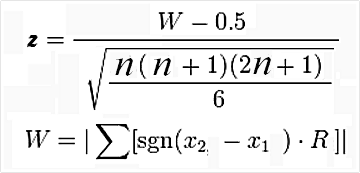# Wilcoxon Signed Rank Test in R

The Wilcoxon Signed Rank Test is the non-parametric equivalent of the t-test. If you have questionswhether or not your data is normally distributed the Wilcoxon Signed Rank Test can still indicate to you if there is a difference between the means of your sample.

Th Wilcoxon Test compares the medians of two samples instead of their means. The differences between the median and each individual value for each sample is calculated. Values that come to zero are removed. Any remaining values are ranked from lowest to highest. Lastly, the ranks are summed. If the rank sum is different between the two samples it indicates statistical difference between samples.

We will now do an example using r. We want to see if there is a difference in enrollment between private and public universities. Below is the code

We will begin by loading the ISLR package. Then we will load the ‘College’ data and take a look at the variables in the “College” dataset by using the ‘str’ function.

``````library(ISLR)
data=College
str(College)``````
``````## 'data.frame':    777 obs. of  18 variables:
##  \$ Private    : Factor w/ 2 levels "No","Yes": 2 2 2 2 2 2 2 2 2 2 ...
##  \$ Apps       : num  1660 2186 1428 417 193 ...
##  \$ Accept     : num  1232 1924 1097 349 146 ...
##  \$ Enroll     : num  721 512 336 137 55 158 103 489 227 172 ...
##  \$ Top10perc  : num  23 16 22 60 16 38 17 37 30 21 ...
##  \$ Top25perc  : num  52 29 50 89 44 62 45 68 63 44 ...
##  \$ F.Undergrad: num  2885 2683 1036 510 249 ...
##  \$ P.Undergrad: num  537 1227 99 63 869 ...
##  \$ Outstate   : num  7440 12280 11250 12960 7560 ...
##  \$ Room.Board : num  3300 6450 3750 5450 4120 ...
##  \$ Books      : num  450 750 400 450 800 500 500 450 300 660 ...
##  \$ Personal   : num  2200 1500 1165 875 1500 ...
##  \$ PhD        : num  70 29 53 92 76 67 90 89 79 40 ...
##  \$ Terminal   : num  78 30 66 97 72 73 93 100 84 41 ...
##  \$ S.F.Ratio  : num  18.1 12.2 12.9 7.7 11.9 9.4 11.5 13.7 11.3 11.5 ...
##  \$ perc.alumni: num  12 16 30 37 2 11 26 37 23 15 ...
##  \$ Expend     : num  7041 10527 8735 19016 10922 ...
##  \$ Grad.Rate  : num  60 56 54 59 15 55 63 73 80 52 ...``````

We will now look at the Enroll variable and see if it is normally distributed

``hist(College\$Enroll)``This variable is highly skewed to the right. This may mean that it is not normally distributed. Therefore, we may not be able to use a regular t-test to compare private and public universities and the Wilcoxon Test is more appropriate. We will now use the Wilcoxon Test. Below are the results

``wilcox.test(College\$Enroll~College\$Private)``
``````##
##  Wilcoxon rank sum test with continuity correction
##
## data:  College\$Enroll by College\$Private
## W = 104090, p-value < 2.2e-16
## alternative hypothesis: true location shift is not equal to 0``````

The results indicate a difference we will now calculate the medians of the two groups using the ‘aggregate’ function. This function allows us to compare our two groups based on the median. Below is the code with the results.

``aggregate(College\$Enroll~College\$Private, FUN=median)``
``````##   College\$Private College\$Enroll
## 1              No       1337.5
## 2             Yes        328.0
``````

As you can see, there is a large difference in enrollment in private and public colleges. We can then make the conclusion that there is a difference in the medians of private and public colleges with public colleges have a much higher enrollment.

Conclusion

The Wilcoxon Test is used for a non-parametric analysis of data. This test is useful whenever there are concerns with the normality of the data.

## 3 thoughts on “Wilcoxon Signed Rank Test in R”

1.Benjam Clarke

Just a short comment regarding the terminology that you use. Are you referring to the Mann Whitney U test here? I know it’s also referred to as Wilcoxon-Mann Whitney or the rank-sum test – the non-parametric test for independent samples (public and private universities in the example given here). I’m not familiar with R though – does it distinguish?